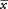## Common Mistakes

It may seem that there are many ways to make errors in working a statistics problem. In fact, most errors on statistics exams can be reduced to a short list of common oversights. If you learn to avoid the mistakes listed here, you can greatly reduce your chances of making an error on an exam.

• Forgetting to convert between standard deviation (σ and s) and variance (σ2 and s2): Some formulas use one; some use the other. Square the standard deviation to get the variance, or take the positive square root of the variance to get the standard deviation.

• Misstating one‐tailed and two‐tailed hypotheses: If the hypothesis predicts simply that one value will be higher than another, it requires a one‐tailed test. If, however, it predicts that two values will be different—that is, one value will be either higher or lower than another or that they will be equal—then use a two‐tailed test. Make sure your null and alternative hypotheses together cover all possibilities—greater than, less than, and equal to.

• Failing to split the alpha level for two‐tailed tests: If the overall significance level for the test is 0.05, then you must look up the critical (tabled) value for a probability of 0.025. The alpha level is always split when computing confidence intervals.

• Misreading the standard normal (z) table: All standard normal tables do not have the same format, and it is important to know what area of the curve (or probability) the table presents as corresponding to a given z‐score. Table 2 in "Statistics Tables" gives the area of the curve lying at or below z. The area to the right of z (or the probability of obtaining a value above z) is simply 1 minus the tabled probability.
• Using n instead of n – 1 degrees of freedom in one‐sample t‐tests: Remember that you must subtract 1 from n in order to get the degrees‐of‐freedom parameter that you need in order to look up a value in the t‐table.
• Confusing confidence level with confidence interval: The confidence level is the significance level of the test or the likelihood of obtaining a given result by chance. The confidence interval is a range of values between the lowest and highest values that the estimated parameter could take at a given confidence level.
• Confusing interval width with margin of error: A confidence interval is always a point estimate plus or minus a margin of error. The interval width is double that margin of error. If, for example, a population parameter is estimated to be 46 percent plus or minus 4 percent, the interval width is 8 percent.

• Confusing statistics with parameters: Parameters are characteristics of the population that you usually do not know; they are designated with Greek symbols (μ and σ). Statistics are characteristics of samples that you are usually able to compute. Although statistics correspond to parameters (is the mean of a sample, as μ is the mean of a population), the two are not interchangeable; hence, you need to be careful and know which variables are parameters and which are statistics. You compute statistics in order to estimate parameters.

• Confusing the addition rule with the multiplication rule: When determining probability, the multiplication rule applies if all favorable outcomes must occur in a series of events. The addition rule applies when at least one success must occur in a series of events.

• Forgetting that the addition rule applies to mutually exclusive outcomes: If outcomes can occur together, the probability of their joint occurrence must be subtracted from the total “addition‐rule” probability.

• Forgetting to average the two middle values of an even‐numbered set when figuring median and (sometimes) quartiles: If an ordered series contains an even number of measures, the median is always the mean of the two middle measures.

• Forgetting where to place the points of a frequency polygon: The points of a frequency polygon are always at the center of each of the class intervals, not at the ends.